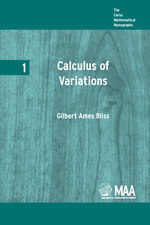Have library access? Log in through your library# Calculus of Variations

Gilbert Ames Bliss
Volume: 1
Copyright Date: 1925
Edition: 1
Pages: 204
https://www.jstor.org/stable/10.4169/j.ctt5hh931

## Table of Contents

1. Front Matter
(pp. i-iv)
2. PREFACE
(pp. v-x)
G. A. Bliss
3. Table of Contents
(pp. xi-xiv)
4. CHAPTER I TYPICAL PROBLEMS OF THE CALCULUS OF VARIATIONS
(pp. 1-16)

When the student of mathematics pauses to look back upon the achievements of mathematicians of the past he must be impressed with the fact that the seventeenth century was a most important epoch in the development of modern mathematical analysis, since to the mathematicians of that period we owe the invention of the differential and integral calculus. At first the calculus theory, if indeed at that time it could be called such, consisted of isolated and somewhat crude methods of solving special problems. In the domain of what we now call the integral calculus, for example, an Italian mathematician named...

5. CHAPTER II SHORTEST DISTANCES
(pp. 17-40)

Problems of determining shortest distances furnish a useful elementary introduction to the theory of the calculus of variations because the properties characterizing their solutions are familiar ones which illustrate very well many of the general principles common to all of the problems suggested in the preceding chapter. If we can for the moment eradicate from our minds all that we know about straight lines and shortest distances we shall have the pleasure of rediscovering well-known theorems by methods which will be helpful in solving more complicated problems.

Let us begin with the simplest case of all, the problem of determining...

6. CHAPTER III THE BRACHISTOCHRONE PROBLEM
(pp. 41-84)

The bracbistochrone problem is historically the most interesting of all the special problems mentioned in Chapter I since as we have there seen it gave the first impetus to systematic research in the calculus of variations. Since the time of the Bernoulli brothers it has been used with great regularity as an illustration by writers on the subject, and it is in many respects a most excellent one. Unfortunately in the forms originally proposed by the Bernoullis it does not require the application of an important necessary condition for a minimum which was first described by Jacobi in 1837, more...

7. CHAPTER IV SURFACES OF REVOLUTION OF MINIMUM AREA
(pp. 85-127)

The problem of determining a surface of revolution of minimum area, like the brachistochrone problem, was one of the earliest considered by students of the calculus of variations, and it is one of those which have been most thoroughly studied. It is in many respects the most satisfactory illustration which we have of the principles of the general theory of the calculus of variations in the plane. In spite of the fact that it was proposed early in the eighteenth century and has been restudied at frequent intervals since that time, one finds nevertheless that new results of interest and...

8. CHAPTER V A MORE GENERAL THEORY
(pp. 128-179)

We have been con sidering in the preceding chapters several problems of the calculus of variations whose integrals to be minimized were all special cases of the more general integral

$I = \int_{{x_1}}^{{x_0}} {f(x,y,y')} dx$

in which the integrand is allowed to contain the variable ÷ as well as the variablesyand${y'}$which have hitherto been present. It is clear that if we can find characteristic properties of minimizing arcs for this integral we shall have results applicable not only to the problems already considered but also to a much larger variety of maximum and minimum questions of the calculus of variations....

9. A LIST OF REFERENCES
(pp. 180-181)
10. NOTES
(pp. 182-184)
11. INDEX
(pp. 187-189)